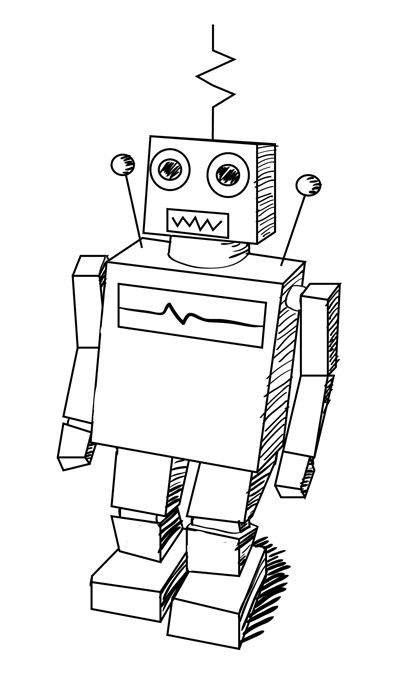# Linear Regression — Intro To Machine Learning #6

Hi folks, last time I wrote about Classification and Regression, by now I expect you to be able to differentiate regression and classification problem. Today we are going to define a regression problem and apply Linear Regression Algorithm as out prediction model.

# What is Regression Problem?

In regression problem the goal of the algorithm is to predict real-valued output.

# Problem Setting

Sales (in thousands of units) for a particular product as a function of advertising budgets (in thousands of dollars) for TV, radio, and newspaper media. Suppose that in our role as statistical consultants we are asked to suggest.

(1). We want to find a function that given input budgets for TV, radio and newspaper predicts the output sales.

(2). Which media contribute to sales?

(3). Visualize the relationship between the features and the response using scatter plots.

# Linear Regression Model

Linear regression is a very simple approach for supervised learning. Though it may seem somewhat dull compared to some of the more modern algorithms, linear regression is still a useful and widely used statistical learning method. Linear regression is used to predict a quantitative response Y from the predictor variable X.

Linear Regression is made with an assumption that there’s a linear relationship between X and Y.

Mathematically, we can write a linear relationship as:

Where:

• y is the response
• β values are called the model coefficients. These values are “learned” during the model fitting/training step.
• β0 is the intercept
• β1 is the coefficient for X1 (the first feature)
• βn is the coefficient for Xn (the nth feature)

# What Happens In The Training Process?

When training a linear regression model it’s way to say we are trying to find out a coefficients for the linear function that best describe the input variables.

When building a linear model it’s said that we are trying to minimize the error an algorithm does making predictions, and we got that by choosing a function to help us measure the error also called cost function.

For that task there’s a mathematical algorithm called Gradient Descent, I’m not going through details now but here is an outline of what it does:

• Keep changing B0 and B1 to reduce the J(B0, B1) until we hopefully end up at a minimum.

# Model evaluation metrics for regression

Evaluation metrics for classification problems, such as accuracy, are not useful for regression problems. Instead, we need evaluation metrics designed for comparing continuous values, here I’m using well Root Mean Squared Error, of course there are others but this is one of the favorites choice and we are going to go along with it.

Root Mean Squared Error is the square root of the mean of the squared errors(MSE), MSE by itself can be used for evaluation metric, but that it’s subject for another post.

# Playground

Want more theory?, no! I got you, I guess you got an intuition of Linear Regression, now let’s have a playground and see linear regression in action:

You can find the data here and it look like this:

Here you can check this plots drawn from this data to inspect the relationship between TV, Radio and Newspaper with Sales.

Here is the code to train Linear Regression Model:

RMSE = 1.40465142303

# Make Experiments == Play with data

If you are using python this statement will be true hahah, bed joke hum, let’s be serious now and make some feature selection.

• Does Newspaper improve the quality of our predictions?
• Hypothesis: Newspaper does not improve model predictions.
• Hypothesis Testing Procedure: Let’s remove Newspaper from the model and check the RMSE (Root Mean Squared Error).
• Error is something we want to minimize, so a lower number for RMSE is better.
• If we wanted to make changes and improvements to a model, the RMSE should be lower if the model is getting better.

RMSE = 1.38790346994

First we got RMSE of 1.40 but after removing Newspaper we got 1.38, What does it mean? Just in case of doubt or you want to share your answer feel free to comment or Tweet me.

Insight: Linear Regression might be old but it’s still useful, but there’s a drawback of using linear regression because it’s made on assumptions that our data have linear relationships while in many real world scenarios that not true. It’s quite useful to understand Linear Regression because of it’s simplicity and later on it will be useful to understand more modern approaches and the state of The art Algorithms such as Neural Networks and many more. Stay tune, will arrive there.

This is all for this post, see you next time, you can find the script and notebook with detailed steps here. If I have missed something in this post or in the code let me know.

In the upcoming post I’ll show you a classification algorithm: Logistic Regression.

Happy Learning.

## Simple AI

Get to know Machine Learning and how to apply the State Of…

## Simple AI

Get to know Machine Learning and how to apply the State Of The Art Algorithms

Written by

## David Fumo

Passionate about technology, software development and financial markets.## Simple AI

Get to know Machine Learning and how to apply the State Of The Art Algorithms